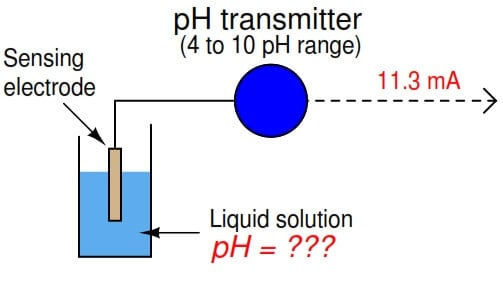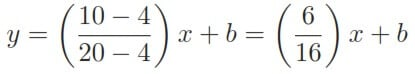# Calculate Process Variable from Transmitter Current

#### A pH transmitter has a calibrated  range  of 4 pH to 10 pH, with a 4-20 mA output  signal. Calculate the pH sensed by the transmitter if its output signal is 11.3 mA.We are solving this problem using standard 4-20mA conversion Formula. Click here for formula

First, we will set up a linear equation describing  this transmitter’s function:Note how we are free to set up 4-20 mA as the independent variable  (x axis) and the pH as the dependent variable (y axis).  We could arrange  current on the y axis and  the process measurement on the x axis as before, but  this would force us to manipulate the linear equation to solve for x.

Calculating and substituting the slope (m)  value for this equation, using the full rise-over-run  of the linear function:We can also solve the problem using direct formula. Click here for formula

#### Articles You May Like :

PID Control terms

Pressure Transmitter Calculations

Relationship of PV, LRV and URV

3-15 psi and 4-20 mA Formula

Temperature Coefficient of RTD

Don't Miss Our Updates
Be the first to get exclusive content straight to your email.
We promise not to spam you. You can unsubscribe at any time.

### 2 thoughts on “Calculate Process Variable from Transmitter Current”

1.Very useful

2.